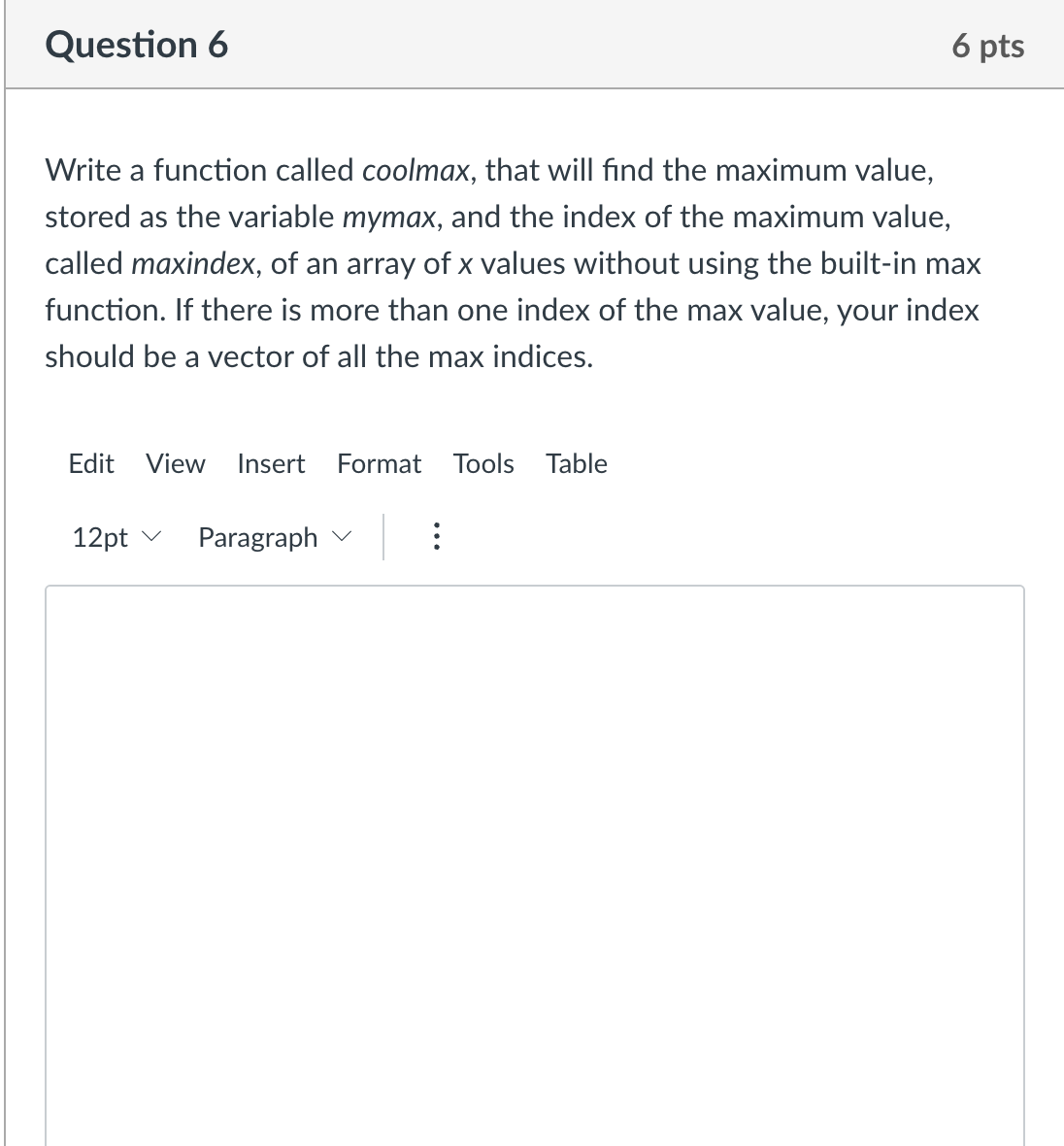# (Solved): MATHLAB PLEase Write a function called coolmax, that will find the maximum value, stored as the var ...Write a function called coolmax, that will find the maximum value, stored as the variable mymax, and the index of the maximum value, called maxindex, of an array of values without using the built-in max function. If there is more than one index of the max value, your index should be a vector of all the max indices.

We have an Answer from Expert

Here's an updated version of the coolmax function in MATLAB that finds the maximum value and the indices of the maximum value in an array x without using the built-in max function:

This implementation iterates over the elements of the input array x and updates mymax and maxindex accordingly. If a larger value is found, mymax is updated, and maxindex is set to the current index. If an element equal to the current maximum value is found, the index is appended to the maxindex vector.

You can use this function by calling it with an array of values, like this:

The output would be:

In this example, the maximum value is 5, and its indices in the array are 2 and 4.
We have an Answer from Expert